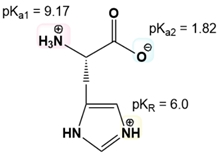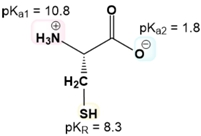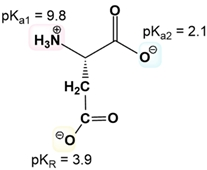Start typing, then use the up and down arrows to select an option from the list.# Biochemistry

Learn the toughest concepts covered in Biochemistry with step-by-step video tutorials and practice problems by world-class tutors

3. Amino Acids

# Isoelectric Point of Amino Acids with Ionizable R-Groups

1

#### concept

Isoelectric Point Of Amino Acids With Ionizable R-Groups12m
Play a video:
2
Problem

What is the pI of His?3
Problem

Electrophoresis separates molecules by migration through a gel only if they have a net charge. How do you expect an amino acid to migrate during electrophoresis when the pH = pI?

4
Problem

Draw Glu and calculate its isoelectric point. pKa1 = 9.67. pKa2 = 2.19. pKR = 4.25.

5
Problem

Calculate Cys’s pI.6
Problem

Calculate the pI of Arg. pKa1 = 9. pKa2 = 2.2. pKR = 12.5.

7
Problem

Calculate the pI of Asp.